# Angle Basics

GCSEKS3Level 1-3AQACambridge iGCSEEdexcelEdexcel iGCSEOCRWJEC

## Angle Basics

Understanding the basics of angles will help you with many other geometry topics. We will cover the different types of angles, angle notation and how to measure angles in this topic.

Level 1-3GCSEKS3AQAEdexcelOCRWJECCambridge iGCSEEdexcel iGCSE

## Skill 1: Types of Angles

There are four types of angles you need to know. These are:

• Right-angles – these are equal to $90\degree$ and are indicated by a square corner.
• Acute angles – these are small angles less than $90\degree$
• Obtuse angles – these are angles larger than $90\degree$ but less than $180\degree$
• Reflex angles – these are the biggest angles and are larger than $180\degree$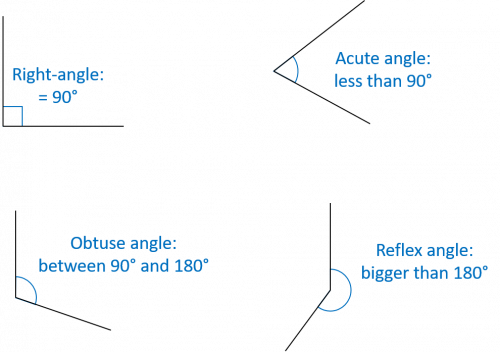Level 1-3GCSEKS3AQAEdexcelOCRWJECCambridge iGCSEEdexcel iGCSE

## Skill 2: Angle Notation

There are a few different ways to label angles, but the most straightforward is to use three letters, where the middle letter indicates the position of the angle. The other two letters indicate the two lines that form the angle.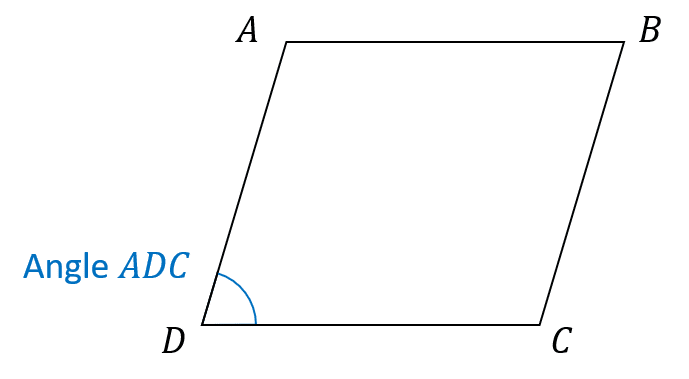For example, in the image shown the angle $\textcolor{#0070C0}{ADC}$ is the angle at the corner $\textcolor{#0070C0}D$ formed by the lines $\textcolor{#0070C0}{AD}$ and $\textcolor{#0070C0}{DC}$.

We could also write this angle as $\textcolor{#0070C0}{\angle ADC}$ or $\textcolor{#0070C0}{A\hat{D}C}$

All three types of notation are perfectly acceptable.

We could also call this angle $\textcolor{#0070C0}{CDA}$. As long as the middle letter is where the angle is and the two other letters indicate the joining lines, they can appear in either order.

Level 1-3GCSEKS3AQAEdexcelOCRWJECCambridge iGCSEEdexcel iGCSE

## Skill 3: Measuring Angles

Measuring angles requires the use of a protractor. Here are some helpful tips for using a protractor:

• Line the middle of the protractor up with the angle ‘corner’
• You need to make sure you are measuring from $0\degree$, so be careful to line things up properly
• Read the scale from $0$ to the exact position on the line to measure the size of the angle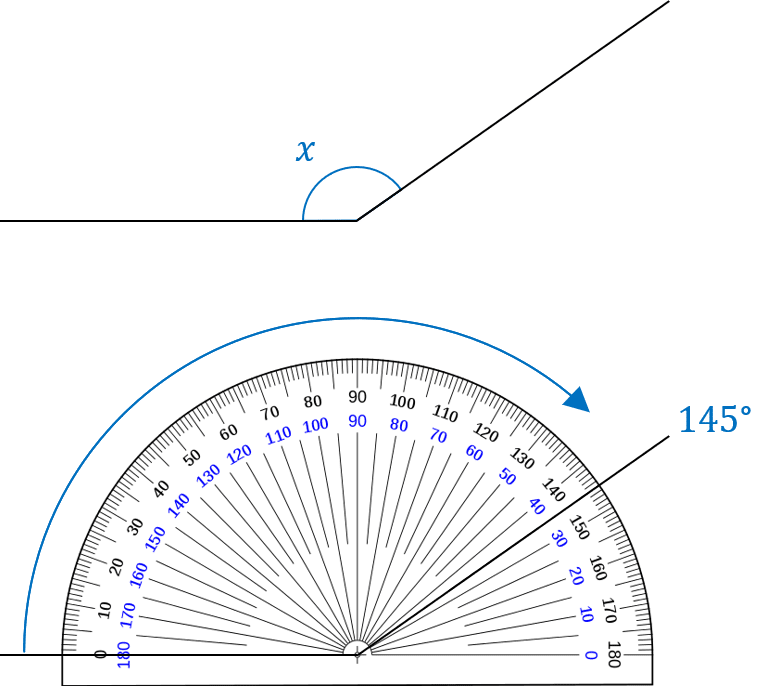Example: Measure the size of the angle marked $\textcolor{#0070C0}x$

Following the steps above, the first thing is to line properly align the protractor. As can be seen, the cross in the centre of the protractor is situated directly over the point or ‘corner’ of the angle $x$.

The protractor is also aligned such that the ‘flat’ line of the angle is at $0\degree$

Reading from $0$, we can see that the other line crosses the scale at $145$, so the angle $\textcolor{#0070C0}{x=145\degree}$

Be careful when using a protractor with two scales, like the one shown – ensure you are always reading from the correct position.

Level 1-3GCSEKS3AQAEdexcelOCRWJECCambridge iGCSEEdexcel iGCSE

## Skill 4: Drawing Angles

Drawing angles requires also requires the use of a protractor. The same tips apply as for measuring angles using a protractor, however the process is slightly different.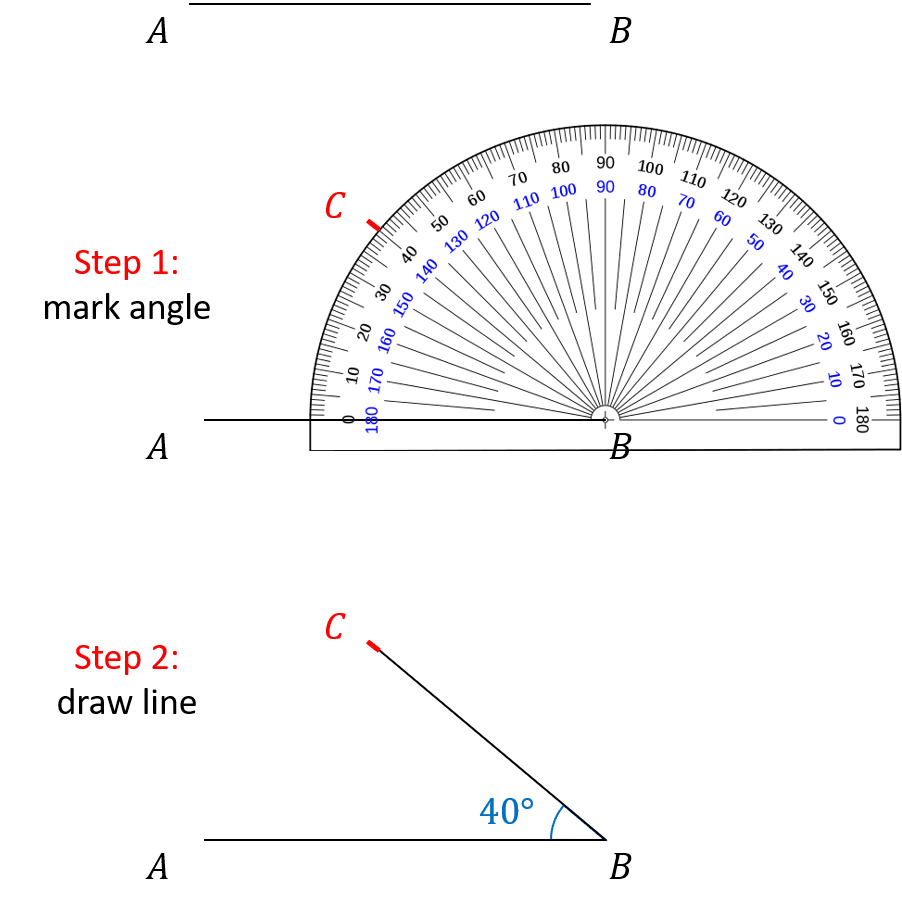Example: Mark another point labelled $C$ such that the angle

$ABC = 40\degree$

The process is similar to measuring an angle but with a couple of differences.

Step 1 is to measure the angle and mark the location of the point. Again, place the centre of the protractor over the point $B$ and align the scale so that the line $AB$ goes through $0\degree$. Mark the point $\textcolor{red}C$ at $40\degree$.

Step 2 is to draw the line between the points $B$ and $C$

Level 1-3GCSEKS3AQAEdexcelOCRWJECCambridge iGCSEEdexcel iGCSE

## Angle Basics Example Questions

Angle $x$ is clearly larger than a right-angle (a square corner), but not as big as the angle in a straight line ($180\degree$). Therefore angle $x$ is obtuse.

Angle $y$ is also obtuse.

Angle $z$ is larger than the angle in a straight line, so it is a reflex angle.Gold Standard Education

Angles $BAD$ and $BCD$ are both equal to $75\degree$

Angles $ABC$ and $ADC$ are both equal to $105\degree$Gold Standard Education

The angle $PQR$ is equal to $60\degree$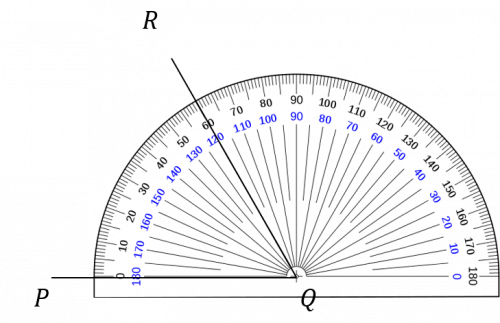Gold Standard Education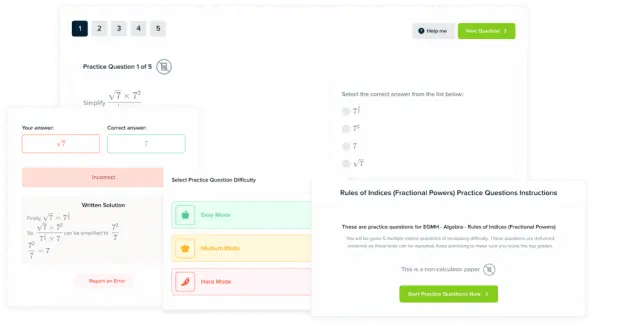Product